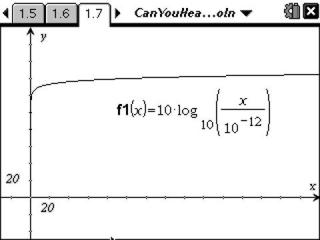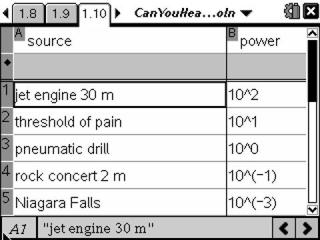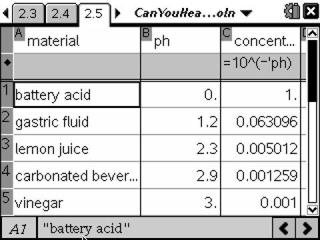# Activities

••• ##### Subject Area

• Math: Precalculus: Logarithmic Functions

• ##### Author9-12

20 Minutes

• ##### Device
• TI-Nspire™
• TI-Nspire™ CAS
• ##### Software

TI-Nspire™
TI-Nspire™ CAS

2.0

• ##### Report an Issue

Can You Hear Me Now?#### Activity Overview

Students will explore logarithmic equations relating to sound intensity and pH.

#### Key Steps

•In this activity, students engage in an exploration of logarithmic functions with real applications. Problem 1 involves the exploration of the relationship between the intensity of sound in W / m2 and the corresponding sound intensity level in decibels (dB). Students observe the large range of values for measured sound intensity and learn that logarithmic functions are helpful in working with data involving large ranges of possible values.

•A spreadsheet is provided with the intensity, or power of sound per square meter, for a variety of situations. Students use this information to calculate the intensity level of sound in decibels (dB).

Students answer a variety of questions involving interpreting given data and application of the concepts involved.

•A second, similar problem is provided as either an extension or for homework. This activity involves the pH scale and students similarly perform calculations in a spreadsheet. Given pH of a variety of common solutions, students are asked to determine the corresponding hydrogen ion concentrations.